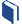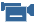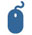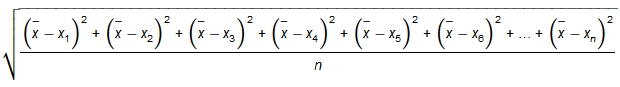# Making Deviation Standard

###Resources for this lesson:

> Glossary> Calculator Resources> Teacher Resources: Instructional NotesAndrew and Khalid are still confused about how to calculate the standard deviation. Justyce suggests they watch a short video for one more example. If you are still confused, take time to watch the video.Sample standard deviation and bias (from Khan Academy)

There is a basic formula for finding the standard deviation. Follow each step below to discover this formula.

• STEP 1: Calculate the mean of the data. The symbol used for mean is x.
• STEP 2: Subtract each data point from the mean, square the differences, and add the differences together. The symbol used for each data point is  for n data points.

##Check Your Understanding

• STEP 3: Divide the sum by the number of data points.

##Check Your Understanding

• STEP 4: Take the square root of the quotient arrived at in Step 3.This is the formula for finding the standard deviation of a set of data.